# WebGL入门(一)：模型变换及其数学基础

## 前言

• Modeling--模型变换
• Viewing--视图变换

1、比如在一个静态模型中，摄像机在移动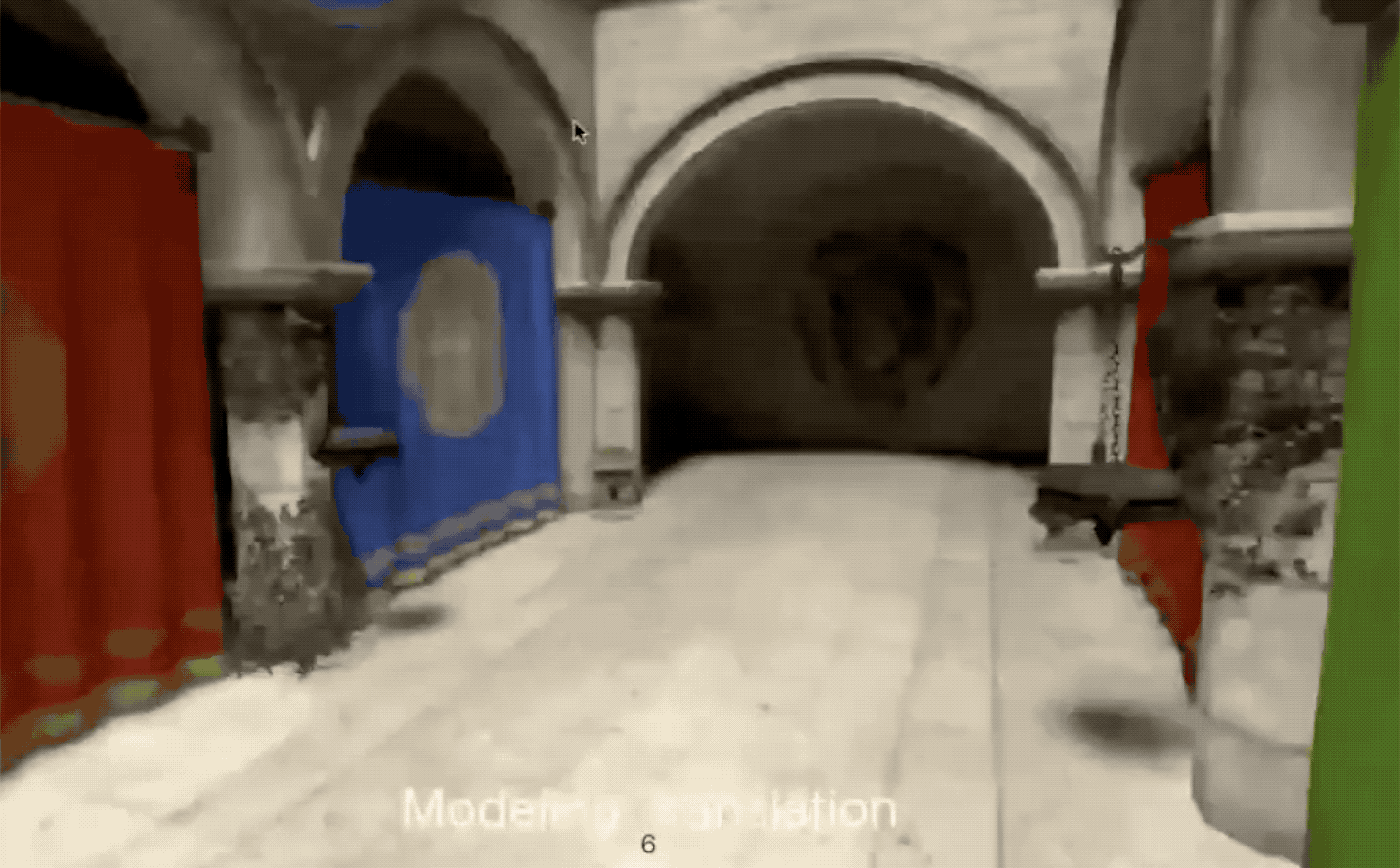2、又比如一个机器人模型在跳舞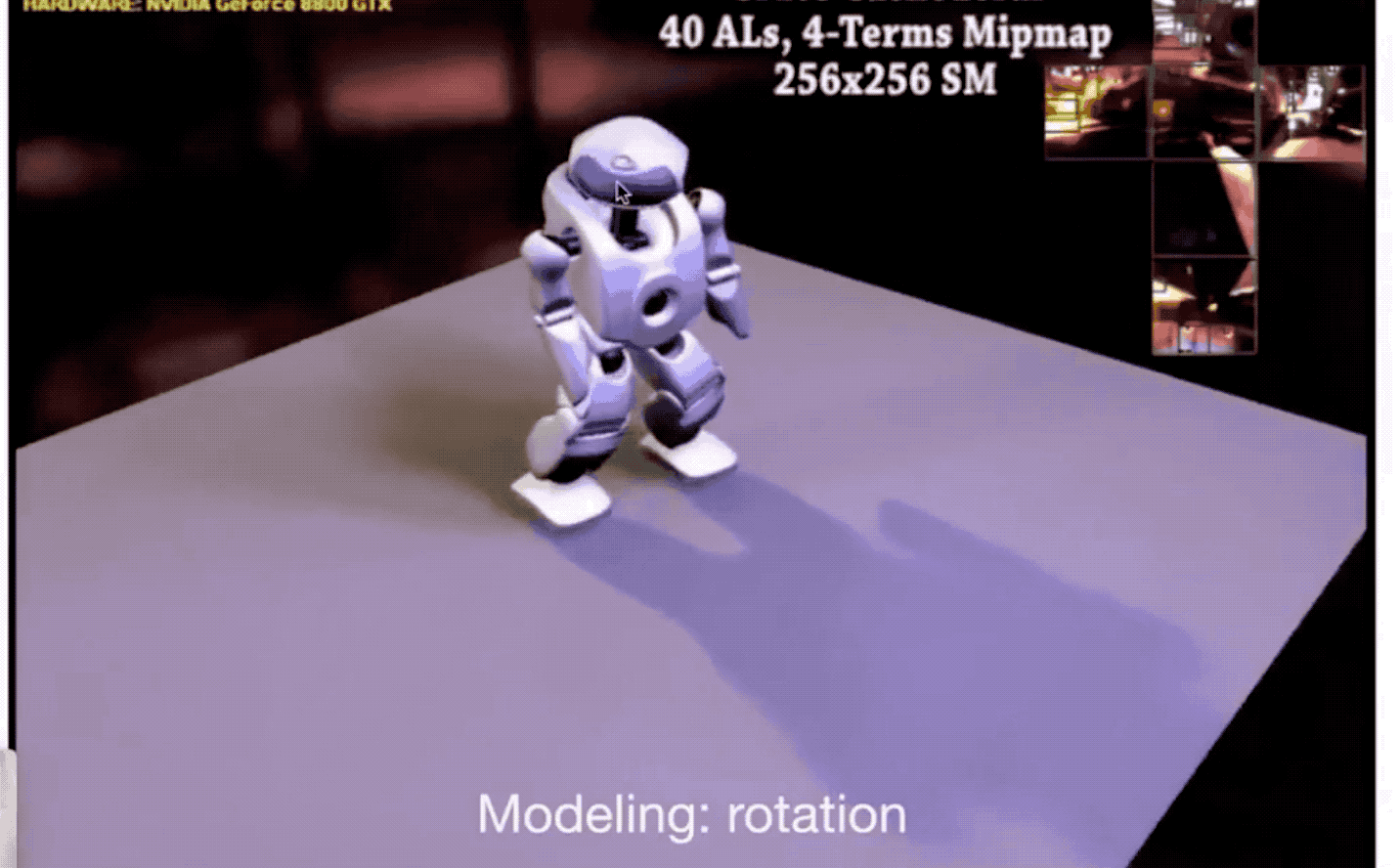## 线性变换

### 线性空间

• 由很多个位置点组成的
• 在空间中可以定义长度、角度和方向等这些概念
• 空间可以存在运动（变换），也就是一个点到另一个点的移动（不是微积分意义上连续的运动）
• ...

$T_{rotate}$就是空间中的运动描述，表述为一个矩阵。

### 向量

1、向量：既有大小又有方向的量，记为$\vec {AB}$或者$\vec a$，在物理上由称为矢量。向量常用有向线段来表示，但也不能说向量就是有向线段，因为向量是“自由的”，可以平移等；而有向线段有固定的起点和终点，不能随便移动。

2、向量的模：向量的大小又叫向量的模，指的是：向量的有向线段的长度。记为:$|\vec {AB}|$或者$|\vec a|$。向量本身不能比大小，只有向量的模才能比大小。

3、零向量：模为0的向量叫零向量，记为$\vec 0$，零向量的特点是方向是任意的，也就是说与所有向量都平行

4、单位向量：模为1个单位长度的非零向量叫单位向量，比如在二维坐标系中，指向正右方的称为$\hat i$，指向正上方的称为$\hat j$$\hat i$$\hat j$被称为二维坐标系的基。

5、向量的表示：根据这两个特殊的基向量构成的坐标系中任一向量$\vec a$都可以表示为$\vec a = x\vec i + y\vec j =$ $\begin{bmatrix} {x}\\ {y}\\ \end{bmatrix}$，此时$|\vec a| = \sqrt {x^2 + y^2} sin\theta$。记住每当用数字描述向量时，它都依赖于正在使用的基。

#### 向量的线性运算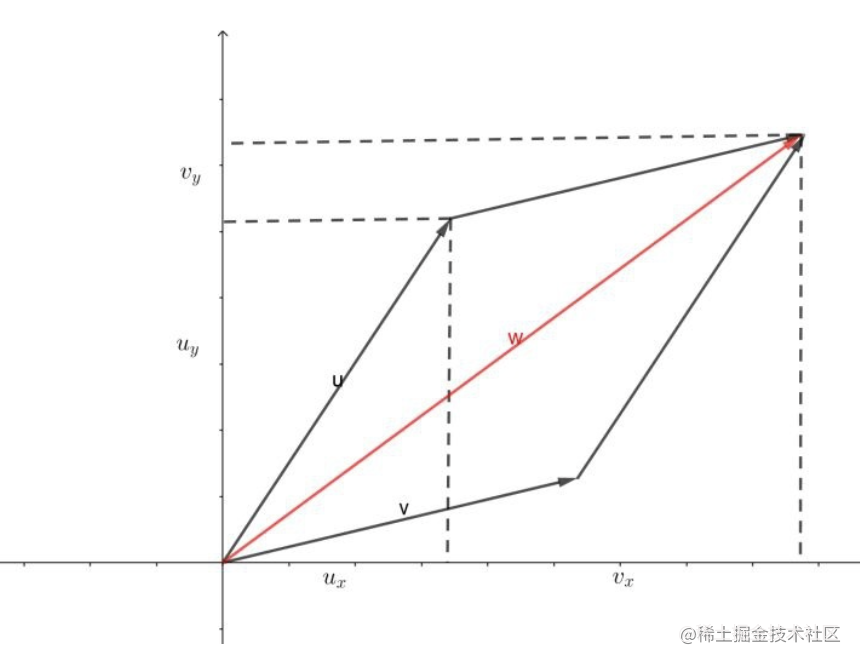1、向量加法

2、向量减法

• 三角形法则
• 平行四边形法则

3、向量点乘

• 已知两个非零向量$\vec a$$\vec b$和它们的夹角$\theta$，则$\vec a * \vec b = |\vec a| * |\vec b| cos\theta$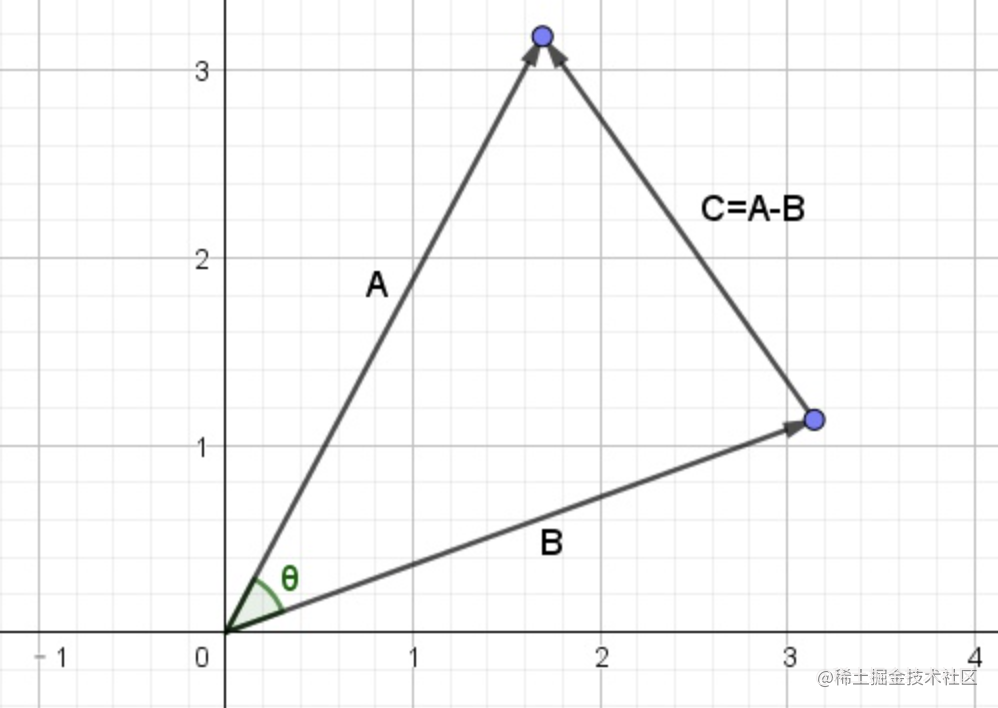证明，利用余弦定理：$|\vec C|^2 = |\vec A|^2 + |\vec B|^2 - 2|A||B|cos \theta$

• 符合交换律、分配律，不符合结合律
• 当角度为90度时，两个向量的点乘为0，这两个向量正交。

1、在二维里，$\vec a * \vec b$ = $\begin{bmatrix} {x_a}\\ {y_a}\\ \end{bmatrix}$ * $\begin{bmatrix} {x_b}\\ {y_b}\\ \end{bmatrix}$ = $x_ax_b + y_ay_b$
2、在三维里，$\vec a * \vec b$ = $\begin{bmatrix} {x_a}\\ {y_a}\\ {z_a}\\ \end{bmatrix}$ * $\begin{bmatrix} {x_b}\\ {y_b}\\ {z_b}\\ \end{bmatrix}$ = $x_ax_b + y_ay_b + z_az_b$

• 找到两个向量间的夹角或者余弦夹角
• 得出一个向量在另一个向量中的投影

• $\vec {b_{\tau}}$的方向一定和$\vec a$是一样的
• 用a表示$\vec a$单位向量，那么$\vec {b_{\tau}} = ka$
• $\vec {b_{\tau}}$$\vec b$组成一个直角三角型

4、向量叉乘

• 判定左和右
• 判定内与外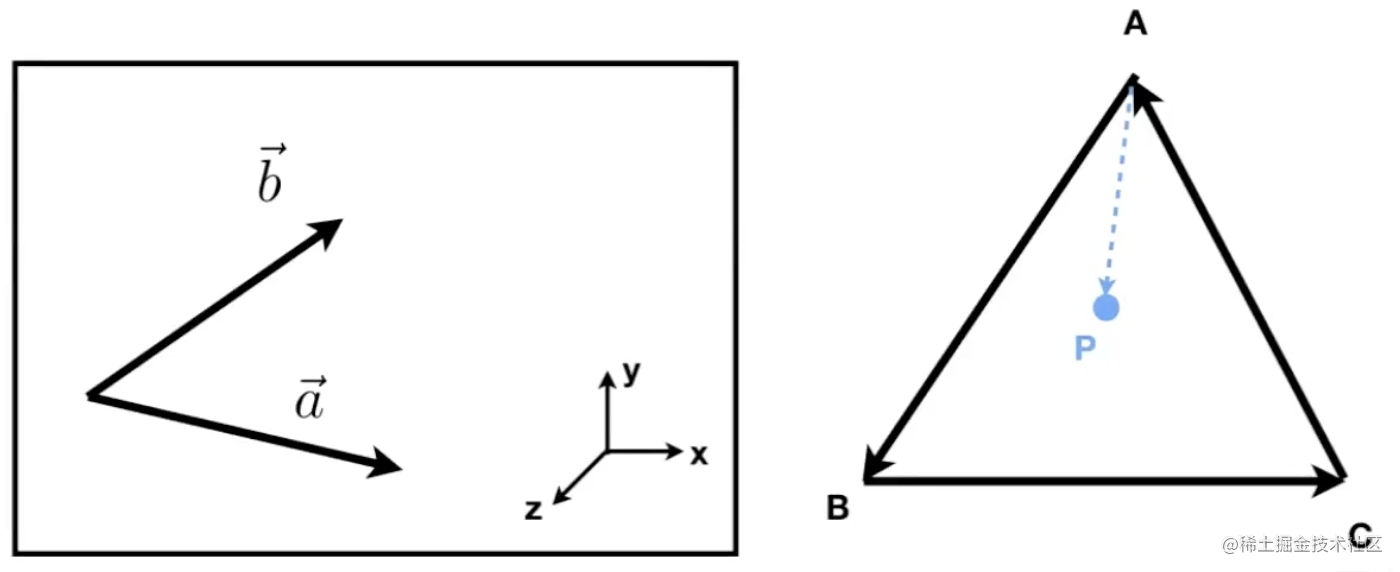比如说左边的图所示，想要知道向量$\vec b$在向量$\vec a$的左边还是右边，或者说向量$\vec a$向逆时针旋转还是顺时针旋转。

• $\vec AB$ x $\vec AP$得出的结果是$\vec AP$$\vec AB$左侧
• $\vec BC$ x $\vec BP$得出的结果是$\vec BP$$\vec BC$左侧
• $\vec CA$ x $\vec CP$得出的结果是$\vec CP$$\vec CA$左侧

### 模型变换

• 直线在变换后仍然保持为直线，不能弯曲
• 原点必须保持固定

#### Scale--缩放变换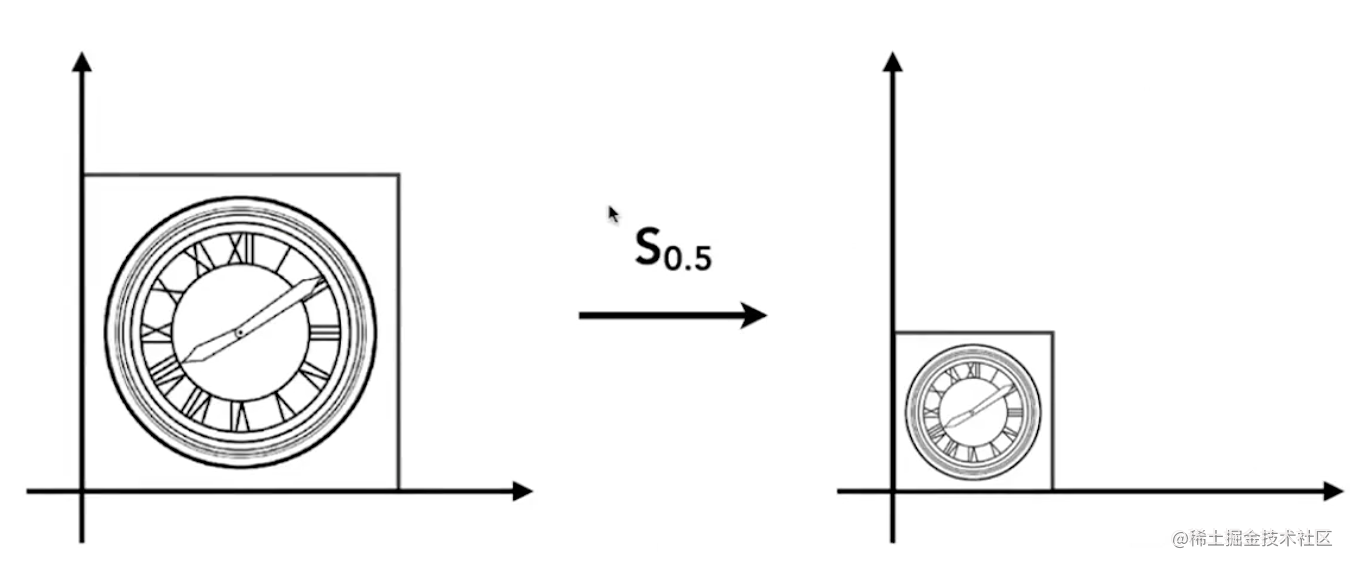如图所示：左边的图形经过$S_{0.5}$的缩放变成右边的图形，因为是在二维坐标系中，所以具体来说就是x轴和y轴都缩放0.5倍。

$x^{'}=sx\\ y^{'}=sy\\$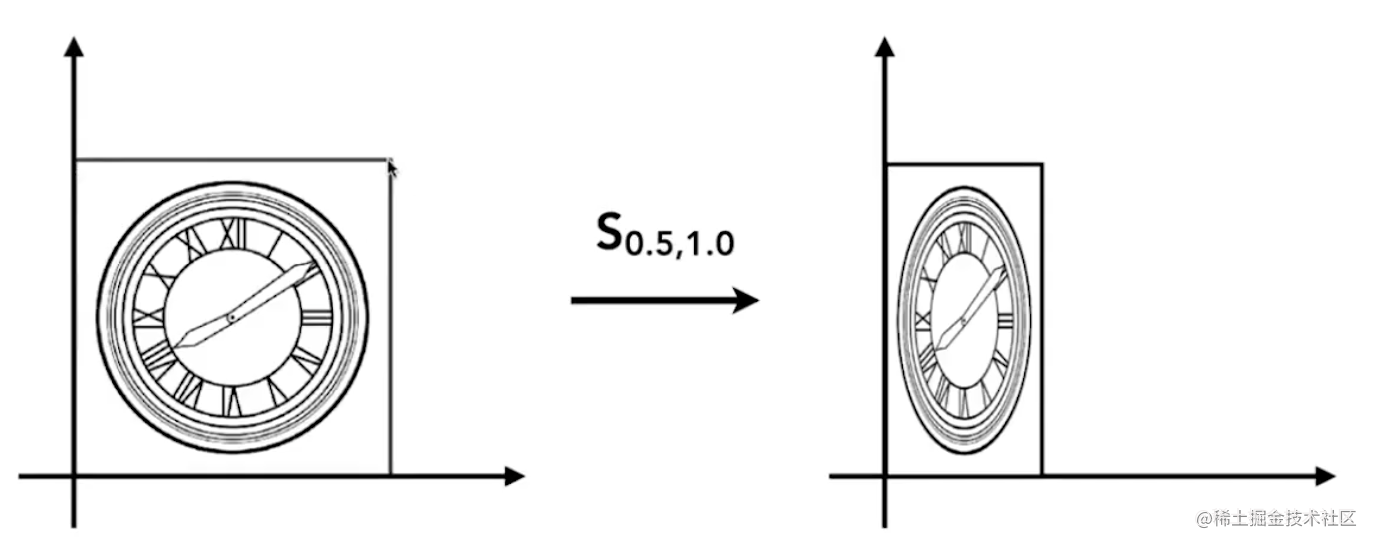如图所示，如果x轴缩放0.5倍y轴不变，那么表达式就变成了$\begin{bmatrix} {x^{'}}\\ {y^{'}}\\ \end{bmatrix}$ = $\begin{bmatrix} {s_{x}}&{0}\\ {0}&{s_{y}}\\ \end{bmatrix}$ $\begin{bmatrix} {x}\\ {y}\\ \end{bmatrix}$

#### Shear--切变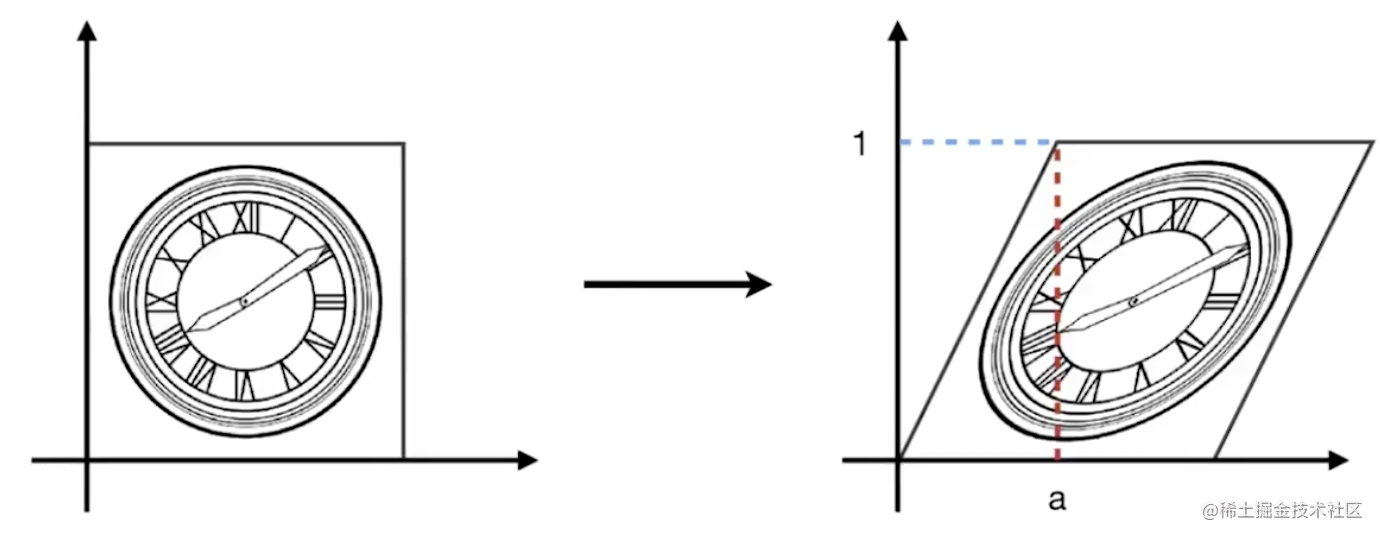如图所示：边长都为1的正方形变换为了一个平行四边形，对比左右图型可以发现：y轴坐标都没有发生变化，图形的下边x轴没有发生变化，只有上边的x轴都加上a，所以中间的点的x左边都要加上ay 列出方程组

$x^{'}=x + ay\\ y^{'}=y\\$

#### Rotate--旋转变换

• 只要不作具体说明，认为都是围绕着原点（0，0）旋转
• 只要不作具体的旋转方向，都认为是逆时针方向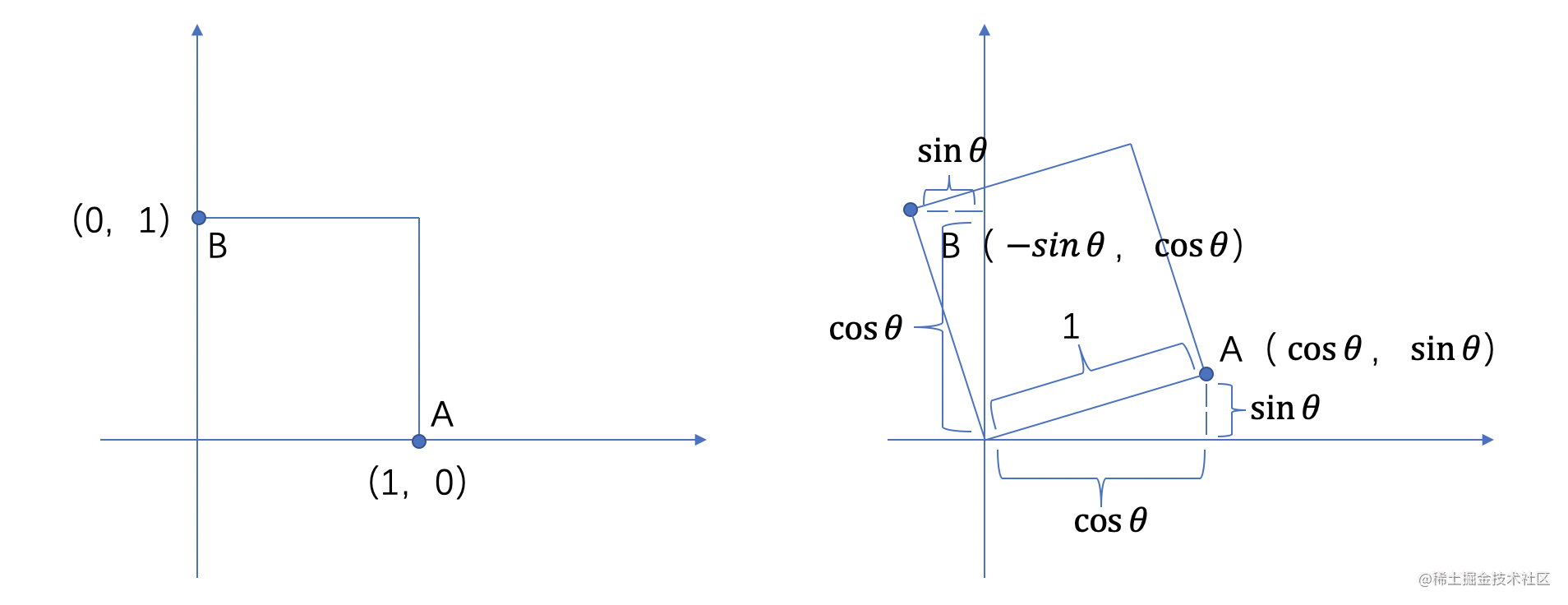如图所示边长为1的正方形，围绕原点(0,0)旋转，旋转$\theta$角度。A点从(1, 0)到$A^{'}(cos \theta，sin \theta)$，B点从(0, 1)到$B^{'}(-sin\theta, cos\theta)$

$x^{'}=cos\theta x - sin\theta y\\ y^{'}=sin\theta x + cos\theta y\\$

$x^{'}=ax + by\\ y^{'}=cx + dy\\$

#### Translation--平移变换

$x^{'}=x + t_x\\ y^{'}=y + t_y\\$

#### 齐次坐标

• 点表示为(x, y, 1)
• 向量表示为(x, y, 0)

1、Scale：$S({s_x, s_y})$ = $\begin{bmatrix} {s_{x}}&{0}&{0}\\ {0}&{s_{y}}&{0}\\ {0}&{0}&{1}\\ \end{bmatrix}$

2、Rotation：$R(\theta$)\$ = $\begin{bmatrix} {cos{\theta}}&{-sin{\theta}}&{0}\\ {sin{\theta}}&{cos{\theta}}&{0}\\ {0}&{0}&{1}\\ \end{bmatrix}$

3、Translation：$T(t_x, t_y)$ = $\begin{bmatrix} {1}&{0}&{t_{x}}\\ {0}&{1}&{t_{y}}\\ {0}&{0}&{1}\\ \end{bmatrix}$

#### 变换组合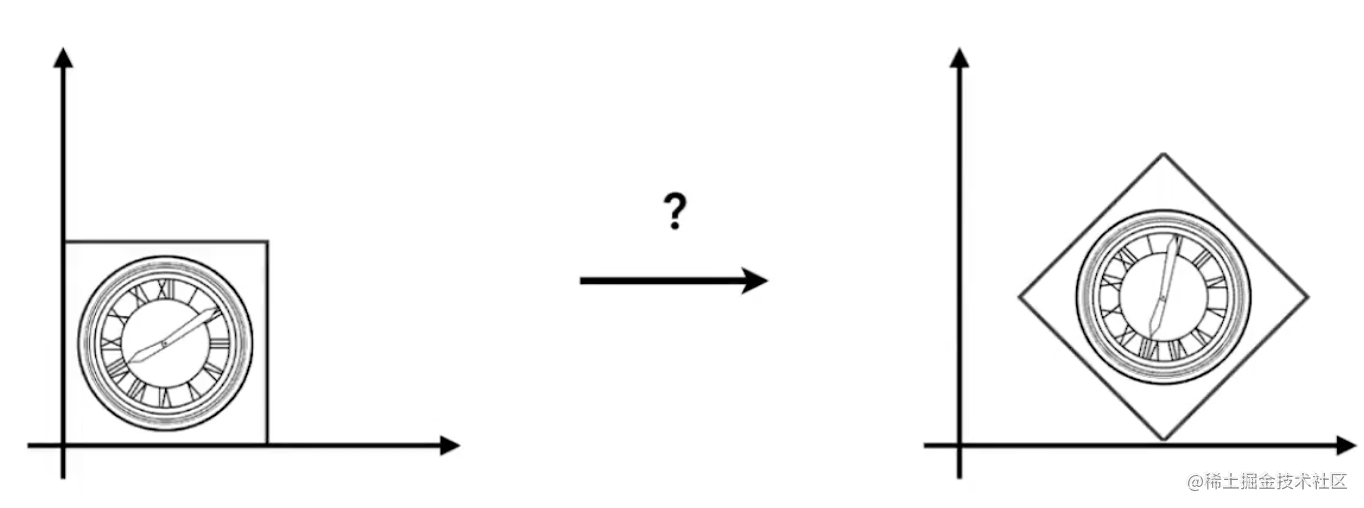如图所示，左边的图怎么变换到右边的图呢？很简单就是平移加旋转，那应该是先平移在旋转还是先旋转再平移？

• 一个复杂的变换能分解成基础变换的组合
• 基础变换的顺序是至关重要的

$T_{(1, 0)} R_{45}$ $\begin{bmatrix} {x}\\ {y}\\ {1} \end{bmatrix}$ = $\begin{bmatrix} {1}&{0}&{1}\\ {0}&{1}&{0}\\ {0}&{0}&{1}\\ \end{bmatrix}$ $\begin{bmatrix} {cos45}&{-sin45}&{0}\\ {sin45}&{cos45}&{0}\\ {0}&{0}&{1}\\ \end{bmatrix}$ $\begin{bmatrix} {x}\\ {y}\\ {1} \end{bmatrix}$

### 三维变换

• 点表示为(x, y, z, 1)
• 向量表示为(x, y, z, 0)

1、Scale：$S({s_x, s_y, s_z})$ = $\begin{bmatrix} {s_{x}}&{0}&{0}&{0}\\ {0}&{s_{y}}&{0}&{0}\\ {0}&{0}&{s_{z}}&{0}\\ {0}&{0}&{0}&{1}\\ \end{bmatrix}$

2、Translation：$T(t_x, t_y)$ = $\begin{bmatrix} {1}&{0}&{0}&{t_{x}}\\ {0}&{1}&{0}&{t_{y}}\\ {0}&{0}&{1}&{t_z}\\ {0}&{0}&{0}&{1}\\ \end{bmatrix}$

（1）：围绕x轴旋转，也就是说x轴坐标不变，y轴与z轴的坐标在做旋转变换，反应到矩阵中：

$R_x(\theta)$ = $\begin{bmatrix} {1}&{0}&{0}&{0}\\ {0}&{cos{\theta}}&{-sin{\theta}}&{0}\\ {0}&{sin{\theta}}&{cos{\theta}}&{0}\\ {0}&{0}&{0}&{1}\\ \end{bmatrix}$

(2)：围绕y轴旋转，同理y轴坐标不变，z轴和x轴坐标做旋转

$R_y(\theta)$ = $\begin{bmatrix} {cos{\theta}}&{0}&{sin{\theta}}&{0}\\ {0}&{1}&{0}&{0}\\ {-sin{\theta}}&{0}&{cos{\theta}}&{0}\\ {0}&{0}&{0}&{1}\\ \end{bmatrix}$

(3)：围绕z轴旋转

$R_z(\theta)$ = $\begin{bmatrix} {cos{\theta}}&{-sin{\theta}}&{0}&{0}\\ {sin{\theta}}&{cos{\theta}}&{0}&{0}\\ {0}&{0}&{1}&{0}\\ {0}&{0}&{0}&{1}\\ \end{bmatrix}$